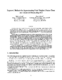## Improved Methods for Approximating Node Weighted Steiner Trees and Connected Dominating Sets##### Files
CS-TR-3849.ps(233.1 KB)
A greedy approximation algorithm based on spider decompositions'' was developed by Klein and Ravi for node weighted Steiner trees. This algorithm provides a worst case approximation ratio of $2 \ln k$, where $k$ is the number of terminals. However, the best known lower bound on the approximation ratio is $\ln k$, assuming that $NP \not\subseteq DTIME[n^{O(\log \log n)}]$, by a reduction from set cover. We show that for the unweighted case we can obtain an approximation factor of $\ln k$. For the weighted case we develop a new decomposition theorem, and generalize the notion of spiders'' to branch-spiders'', that are used to design a new algorithm with a worst case approximation factor of $1.5 \ln k$. This algorithm, although polynomial, is not very practical due to its high running time; since we need to repeatedly find many minimum weight matchings in each iteration. We are able to generalize the method to yield an approximation factor approaching $1.35 \ln k$. We also develop a simple greedy algorithm that is practical and has a worst case approximation factor of $1.6103 \ln k$. The techniques developed for the second algorithm imply a method of approximating node weighted network design problems defined by 0-1 proper functions. These new ideas also lead to improved approximation guarantees for the problem of finding a minimum node weighted connected dominating set. The previous best approximation guarantee for this problem was $3 \ln n$. By a direct application of the methods developed in this paper we are able to develop an algorithm with an approximation factor approaching $1.35 \ln n$. (Also cross-referenced as UMIACS-TR-97-80)